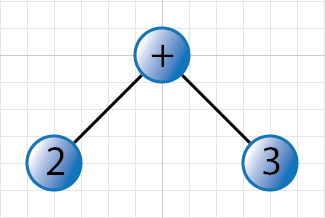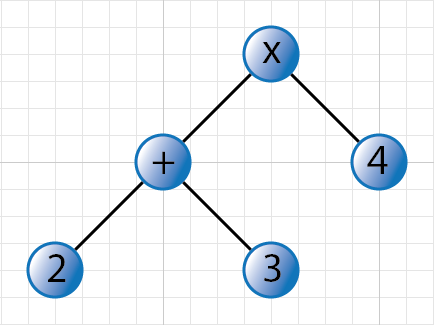## PostFix to Infix: converting RPN to algebraic expressions

Yesterday afternoon I finished my latest PCPlus article on generating all possible arithmetic expressions with four operators. The article explored several algorithms, such as evaluating all full binary trees with a certain number of internal nodes (mine was four), evaluating an expression tree, and the like, and for the sidebar I slipped in a quick bit about RPN (Reverse Polish Notation) and how succinct it is for describing arithmetic expressions (there’s no operator precedence or parentheses to worry about). Equally important is the absolute ease with which you can evaluate an RPN expression compared to an algebraic one (that is, an expression using the standard infix notation).I sent it off but then started to think about how to convert an RPN expression (say, `1 2 3 4 5 + × - ÷`) into its algebraic equivalent (`1 ÷ (2 - 3 × (4 + 5))`, or 0.04). The reason for doing this is to keep and use the speedy succinct RPN form internally, but to display any result in the normal format. There’s lots of information online about doing the reverse operation — that is, converting infix to postfix, for example Dijkstra’s Shunting-yard algorithm, which I first implemented at university using FORTRAN (shudder) — but virtually none about doing the opposite (and some of them I’ve seen even assume that the postfix expression has parentheses, for heaven’s sake).

So, as I said, I thought about it for a while and came up with this answer.

The easy algorithm is to read the RPN expression as if you were going to evaluate it but build up an algebraic expression instead.

Let’s show this with `2 3 + 4 ×`. We read the 2 and push it onto the stack. We do the same with the 3. The next token is +, which we process by popping the right-hand operand (call this the rhs for right hand side), the left hand operand (lhs), forming an algebraic expression with parentheses: `(2 + 3)` and then pushing it onto the stack. Push the next token, the 4. The next token is ×. We pop the rhs (the 4), the lhs (the bracketed expression), form a new expression as before: `((2 + 3) × 4)`, and push it onto the stack. There’s no more RPN expression left, and so the answer is the final string on the stack. Not too bad, expect for the superfluous parentheses around the entire answer.

The problem with this simple algorithm is that it pays no attention to precedence and to avoid any problems with it surrounds each sub-expression with parentheses. For example, `2 3 × 4 +` would be converted to `((2 × 3) + 4)`, instead of the more succinct, yet unambiguous `2 × 3 + 4`.

So how can we improve on the situation? First of all we assign a precedence number to each of the four arithmetic operators: add and subtract get 1, and multiply and divide get 2. That way we can easily say that, for example, multiply is of greater precedence than addition (that is, given a choice between multiply and divide, we do the multiplication first).

We’re going to build up an expression tree from the RPN expression instead of a simple string as in the previous algorithm. We proceed as before but now the stack will store nodes of the expression tree.

Let’s show this with `2 3 + 4 ×` again. We read the 2, create a node to hold it, and push that node onto the stack. We do the same with the 3. The next token is +, which we process by popping the rhs, the lhs, and form a mini tree with a new root node to hold the +.We push the root node, the operand node, onto the stack. We then read the 4, so create a node for it, and push that. The next token is ×. For this we pop the rhs, then the lhs, and form a new tree with the × operator as root:This new root gets pushed onto the stack. As before, we’ve run out of RPN expression and so the answer is the top item on the stack. Of course, we’re not quite there yet: an expression tree is not an algebraic expression, but we’re close.

We now walk the tree using an inorder traversal, paying attention to precedence. In fact we use a rule that says if we go from an operator with a higher precedence to one with a lower precedence, we’ll output parentheses to surround the subexpression containing the lower precedence operator. If the precedence number is equal or lower, we don’t output any parentheses. Here’s the pseudo code:

```string Visit(node, priorPrecedence) {
if node is number
return number.ToString()
result = Visit(left, node.precedence) +
node.Operator +
Visit(right, node.precedence)
if node.precedence < priorPrecedence
result = '(' + result + ')'
return result
}```

There are some assumptions here but they’re pretty obvious (for example, there’s some way to distinguish a number node from an operator node, operator nodes have left and right children, and so on).

So, a two-step process, but nothing too difficult once you’re used to the stack-based approach to evaluating an RPN expression. I quite like this idea of reconstructing an expression tree and then traversing it in a different order than before.Now playing:
Sweetback - Round And Round
(from Stage (2))Loading links to posts on similar topics...

#### 9 Responses##### #1MattiasA said...
19-May-10 7:48 AM

3 2 1 - -

Parens aren't always unnecessary.##### #2julian m bucknall said...
24-May-10 9:57 AM

MattiasA: Sorry, but your concise comment verges on the cryptic. I have no idea what you're trying to say. That RPN expressions are hard to read? Sure, I'll grant you that, but using RPN expressions internally to an application for speed/ease-of-evaluation reasons should not be held back by a human need to read. (Besides which an RPN expression would best be represented by a linked list of nodes and not a space-delimited string such as that I've used.)

Cheers, Julian##### #3MattiasA said...
31-May-10 10:37 PM

Sorry for being overly terse. What I mean is this:

"3 2 1 - -" reduces to "3 1 -", which evaluates to "2".

It looks to me like your algorithm turns "3 2 1 - -" into "3 - 2 - 1". (Because "-" has the same precedence as "-", it omits the parens.) "3 - 2 - 1" reduces to "1 - 1", which evaluates to "0".

The input RPN expression does not have the same value as the output in-order expression, indicating a bug.

The bug is that the parens are not superfluous, in this case, because they change the order of operations (left-to-right; right-to-left) among operations having the same "precedence".

To properly handle situations like this, you'd have to fix the algorithm. The simple solution is to just add more parens--however, since you specifically call this "[t]he problem with this simple algorithm", I presume you'd rather take a different approach.

(Or am I missing something?)##### #4julian m bucknall said...
02-Jun-10 4:06 PM

MattiasA: Very nice catch indeed! I doff my hat. I hadn't considered this particular scenario at all.

In fact, the same problem occurs with repeated division as well: the algebraic expression without parens would assume left to right evaluation. Starting with 2 3 4 / / (answer 2.667), you'd get 2/3/4 (answer 0.167). Time to rethink a little.

Cheers, Julian##### #5Alexandros Katechis said...
03-May-11 10:08 AM

May I suggest a fix to the above bug:

It seems as if when the above bug happens, the tree contains the expression with a higher precedence in the right hand side of the tree. So what I did to fix this (in the limited set of test cases I had) was:

After traversing left and right, check if the rhs is an expression (instead of simply a number), and if it has not already been wrapped in parentheses, wrap it.

I have not done extensive testing of this, so proceed with caution if implementing this. The updated pseudo code would be something like this:

```string Visit(node, priorPrecedence) {
if node is number
return number.ToString()
lhs = Visit(left, node.precedence)
rhs = Visit(right, node.precedence)
if right node is not a number and does not begin with '('
rhs = '(' + rhs + ')'
result =  lhs + node.Operator + rhs
if node.precedence < priorPrecedence
result = '(' + result + ')'
return result
}```

(Fixed code as pre block by Julian.)

##### #6kharen said...
12-Feb-13 6:20 PM

can you help me guys to do a program? its about converting Postfix to Infix Notation.##### #7julian m bucknall said...
12-Feb-13 7:39 PM

@kharen: If this post plus the following one don't explain the situation enough for you to write such a program, there isn't a lot I can do.

Cheers, Julian##### #8Abhay said...
19-Nov-16 11:34 AM

How to write infix of 210+ which is derived from 2+10##### #9julian m bucknall said...
19-Nov-16 1:36 PM

Abhay: Well the issue is that `210+` is an ambiguous postfix expression. Does it mean `+` (as you're assuming) or does it mean `+`? With the information that we have, we cannot know. Therefore, I'd have to say that the language we use to define our postfix expressions has to be "improved", much as we use parentheses in an infix world. In my preamble just now I used square brackets to define the values in my two postfix alternatives.

In other words, if you are converting an infix expression like `2+10` into a postfix version, you would have to use square brackets (or in code, I'd say you'd declare some kind of "number" class with a value property), and then instantiate an instance of it as you parse a number.

Cheers, Julian

##### Leave a response

Note: some MarkDown is allowed, but HTML is not. Expand to show what's available.

•  Emphasize with italics: surround word with underscores `_emphasis_`
•  Emphasize strongly: surround word with double-asterisks `**strong**`
•  Link: surround text with square brackets, url with parentheses `[text](url)`
•  Inline code: surround text with backticks ``IEnumerable``
•  Unordered list: start each line with an asterisk, space `* an item`
•  Ordered list: start each line with a digit, period, space `1. an item`
•  Insert code block: start each line with four spaces
•  Insert blockquote: start each line with right-angle-bracket, space `> Now is the time...`